Contemporary Mathematics

# 6.8The Basics of Loans

Contemporary Mathematics6.8 The Basics of Loans

Figure 6.17 Loans are contracts that allow people to buy now but require them to pay more. (credit: "Closing" by Tim Pierce/Flickr, CC BY 2.0)

### Learning Objectives

After completing this section, you should be able to:

1. Describe various reasons for loans.
2. Describe the terminology associated with loans.
3. Understand how credit scoring works.
4. Calculate the payment necessary to pay off a loan.
6. Determine the cost to finance for a loan.

New car envy is real. Some people look at a new car and feel that they too should have a new car. The search begins. They find the model they want, in the color they want, with the features they want, and then they look at the price. That’s often the point where the new car fever breaks and the reality of borrowing money to purchase the car enters the picture. This borrowing takes the form of a loan.

In this section, we look at the basics of loans, including terminology, credit scores, payments, and the cost of borrowing money.

### Reasons for Loans

Even if you want a new car because you need one, or if you need a new computer since your current one no longer runs as fast or smoothly as you would like, or you need a new chimney because the one on your house is crumbling, it’s likely you do not have that cost in cash. Those are very large purchases. How do you buy that if you don ’t have the cash?
You borrow the money.

And for helping you with your purchase, the company or bank charges you interest.

Loans are taken out to pay for goods or services when a person does not have the cash to pay for the goods or services. We are most familiar with loans for the big purchases in our lives, such as cars, homes, and a college education. Loans are also taken out to pay for repairs, smaller purchases, and home goods like furniture and computers.

Loans can come from a bank, or from the company selling the goods or providing the service. The borrower agrees to pay back more than the amount borrowed. So there is a cost to borrowing that should be considered when deciding on a purchase bought with credit or a borrowed money.
Even using a credit card is a form of a loan.

Essentially, a loan can be obtained for just about any purchase, large or small, that has a cost beyond a person’s cash on hand.

### The Terminology of Loans

There are many words and acronyms that get used in relation to loans. A few are below.

APR is the annual percentage rate. It is the annual interest paid on the money that was borrowed. The principal is the total amount of the loan, or that has been financed. A fixed interest rate loan has an interest rate that does not change during the life of the loan. A variable interest rate loan has an interest rate that may change during the life of the loan. The term of the loan is how long the borrower has to pay the loan back. An installment loan is a loan with a fixed period, and the borrower pays a fixed amount per period until the loan is paid off. The periods are almost uniformly monthly. Loan amortization is the process used to calculate how much of each payment will be applied to principal and how much is applied to interest. Revolving credit, also known as open-end credit, is how most credit cards work but is also a kind of loan account. (We will learn about credit cards in Credit Cards) You can use up to some specified value, called the limit, any way you want, and as long as you pay the issuer of the credit according to their terms, you can keep borrowing from this account.

These and other terminologies can be researched further at Forbes.

### Who Knew?

#### Credit Scores

Not everyone pays the same interest for the same loan. One person might get an APR of 2.9% while another pays 6.9%. These rates are based on your credit score.

Data about you and your credit is collected by three credit bureaus—Experian, Equifax, and TransUnion. They calculate your score using one of two main models: FICO and Vantage Score. The score they develop is based on the following categories:

• Payment History: Making your payments on time and not missing payments is by far the most important factor. All three credit types—revolving, installment, and open—contribute to this factor.
• Credit Utilization or Amount Owed: How much do you owe on your credit card accounts? This category is concerned with the ratio of how much you owe on revolving credit accounts relative to your available credit, also known as your credit utilization ratio.
This is the only category that depends solely on your revolving credit accounts.
• Length of Credit History: This is the average age of your credit history, including the age of the oldest and newest accounts. All three types of credit accounts play a role in this category.
• Credit Mix: This number represents the different types of credit accounts you have, such as credit cards, car loan, mortgages, and whether you are successful managing both revolving and installment accounts.
• New Credit: Have you recently opened a new account or applied for new credit? Lenders want to know how much new credit you are taking on. So, if you are planning to buy a car and make another large purchase with a credit card, you may want to space these purchases out.

If you have done well in these categories, your credit score will be high, and you will qualify for lower interest rates because you are not perceived as being a risky investment. However, if you do poorly in these categories, your score will be low and you will pay higher interest rates since you present a greater risk.

### Who Knew?

#### Where Do Interest Rates Come From?

There are many factors that impact your interest rate beyond your credit score. Banks have the authority to set their own rates, so competition between banks impacts interest rates. Bank A doesn’t want to charge interest rates that are too high, since borrowers will find banks with better rates. Banks also don’t want to charge too little interest.

The too little interest is more involved than the too high. The bank needs to make a profit on its loans. Deposits at the bank are used by the bank to generate loans. The bank has to pay those depositors interest. The bank must charge more for loans they give than they pay to people with deposits in the bank.

Banks also borrow money from each other. These loans have an interest rate, and once more, the bank making a loan must make a profit. More directly, they must charge more for loans they give than they pay for loans they take. In the United States, banks may also borrow from the Federal Reserve, which also charges an interest rate, which is also called the discount rate.

This is where a bank’s prime rate comes from. A bank’s prime rate is the interest rate it will give to its very best customers, which means most customers will pay more than the bank’s prime rate. To confuse the issue, there is also the Wall Street Journal’s prime rate. It is the average of the prime rates charged by individual banks. The Wall Street Journal surveys several banks to generate this value.

Banks also increase the interest rate charged to customers based on both the credit risk presented by the customer, and the risk associate with what the loan will be used for.

### Calculating Loan Payments

Loan payments are made up of two components. One component is the interest that accrued during the payment period. The other component is part of the principal. This should remind you of partial payments from Simple Interest.

Over the course of the loan, the amount of principal remaining to be paid decreases. The interest you pay in a month is based on the remaining principal, just as in the partial payments of Simple Interest.

### FORMULA

The amount of interest, $II$, to be paid for one period of a loan with remaining principal $PP$ is $I=P×rnI=P×rn$, where $rr$ is the interest rate in decimal form and $nn$ is he number of payments in a year (most often $nn$ = 12). Since the interest is for the one period, the time is 1 and does not impact the calculation. Note, interest paid to lenders is always rounded up to the next penny.

### Example 6.77

#### Interest for a Monthly Payment of a Loan

Find the interest to be paid for the period on loans with the following remaining principal and given annual interest rate. Each period is a month.

1. Remaining principal is $13,450, interest rate is 6.75% 2. Remaining principal is$8,460, interest rate is 5.99%

Find the interest to be paid for the period on loans with the following remaining principal and given annual interest rate. Each period is a month.
1.
Remaining principal is $56,945, interest rate is 7.5% 2. Remaining principal is$25,850, interest rate is 2.9%

The payment of the loan has to be such that the principal of the loan is paid off with the last payment. In any period, the amount of interest is defined by the formula above, but changes from period to period since the principal is decreasing with each payment. The trick is knowing how much principal should be paid each payment so that the loan is paid off at the stated time. Fortunately, that is found using the following formula.

### FORMULA

The payment, $pmtpmt$, per period to pay down a loan with beginning principal $PP$ is $pmt=P×(r/n)×(1+r/n)n×t(1+r/n)n×t−1pmt=P×(r/n)×(1+r/n)n×t(1+r/n)n×t−1$, where $rr$ is the annual interest rate in decimal form, $tt$ is the number of years of the loan, and $nn$ is the number of payments per year (typically, loans are paid monthly making $nn$ = 12).

Note, payment to lenders is always rounded up to the next penny.

### Checkpoint

Often, the formula takes the form $pmt=P×(r)×(1+r)n(1+r)n−1pmt=P×(r)×(1+r)n(1+r)n−1$, where $rr$ is the interest rate per period (annual rate divided by the number of periods per year), and $nn$ is the total number of payments to be made.

### Example 6.78

#### Calculating the Payment for a Loan

In the following, calculate the payment necessary to pay off the loan with the given details. The payments are monthly.

1. A car loan taken out for $28,500 at an annual interest rate of 3.99% for 5 years. 2. A home loan taken out for$136,700 and an annual interest rate of 5.75% for 15 years.

In the following, calculate the payment necessary to pay off the loan with the given details. The payments are monthly.
1.
A home improvement loan taken out for $17,950 at an annual interest rate of 7.5% for 10 years. 2. A solar panel installation loan taken out for$33,760 and an annual interest rate of 4.3% for 20 years.

### Tech Check

#### Using Google Sheets to Calculate Loan Payments

Google Sheets has a formula to calculate the monthly payment necessary to pay off a loan with a specified interest rate and term. The formula is the PMT formula. It uses the principal $PP$, interest rate $rr$, and $tt$ years. Follow these steps

Open a Google worksheet and click on any cell.

Type =-PMT(r/12,12*t,P).

Hit the enter key.

The cell displays the payment.

Please note the negative sign. Since Google Sheets is a spreadsheet program, is sees the payment as funds leaving the account, and so they are, by default, negative. The negative sign in front of the formula makes the result positive.

For example, for a $50,000 loan at 10.9% interest for 7 years, you would type =-PMT(0.109/12,12*7,50000). The formula and the result are shown in Figure 6.18. Figure 6.18 Google Sheets formula Alternatively, you can also use an online calculator to find monthly payments for a loan, such as the one at Caluculator.net ### Reading Amortization Tables An amortization table or amortization schedule is a table that provides the details of the periodic payments for a loan where the payments are applied to both the principal and the interest. The principal of the loan is paid down over the life of the loan. Typically, the payments each period are equal. Importantly, one of the columns will show how much of each payment is used for interest, another column shows how much is applied to the outstanding principal, and another column shows the remaining principal or balance Figure 6.19. Figure 6.19 Amortization table ### Example 6.79 #### Reading from an Amortization Table Using the partial amortization table (Figure 6.23), answer the following questions. Figure 6.20 Amortization table 1. What is the loan amount (principal), the interest rate, and the term of the loan? 2. How much is the monthly payment? 3. How much remaining balance is there after the payment in month 15? 4. How much was the interest in payment 10? 5. What is the total of the interest paid after payment 18? 6. What happens to the amount paid in interest each month? ### Your Turn 6.79 Using the partial amortization table below, answer the following questions. Amortization table 1. What is the loan amount (principal), the interest rate, and the term of the loan? 2. How much is the monthly payment? 3. How much remaining balance is there after the payment in month 20? 4. How much was the interest in payment 5? 5. What is the total of the interest paid after payment 24? 6. What happens to the amount paid to the principal each month? ### Video ### Cost of Finance There are often costs associated with a loan beyond the interest being paid. The cost of finance of a loan is the sum of all costs, fees, interest, and other charges paid over the life of the loan. ### Example 6.80 #### Cost of Financing a Personal Loan Irena signed for a loan of$15,000 at 6.33% for 5 years. When she took out the loan, Irena paid a $750 origination fee. Over the course of the loan, she pays$2,537.96 in interest. What was her cost to finance the loan?

1.
Samantha takes out a $28,000 loan for 6 years at 5.5% interest. When she took out the loan, she paid$4,937.18 in interest, an origination fee of $1,400 and an application fee of$100. What was her cost of finance for this loan?

48.
Give three reasons for taking out a loan.
49.
What is an installment loan?
50.
In an installment loan what happens to the amount of the payment that is applied to interest in later payments compared to earlier payments?
51.
An installment loan is paid monthly. The remaining principal is $5,498. If the interest rate is 12.9%, how much interest is paid that month? 52. A business loan is taken out for$250,000 for 15 years at 6.9% interest. What are the payments for the loan?
53.
Consider the amortization table below. What is the balance after the eleventh payment?
54.
The total interest paid on a loan is $38,519.50. The origination fee was$300 while the processing fee was $250. An additional fee was charged to the borrowers, amounting to another$475. How much was the cost of financing for this loan?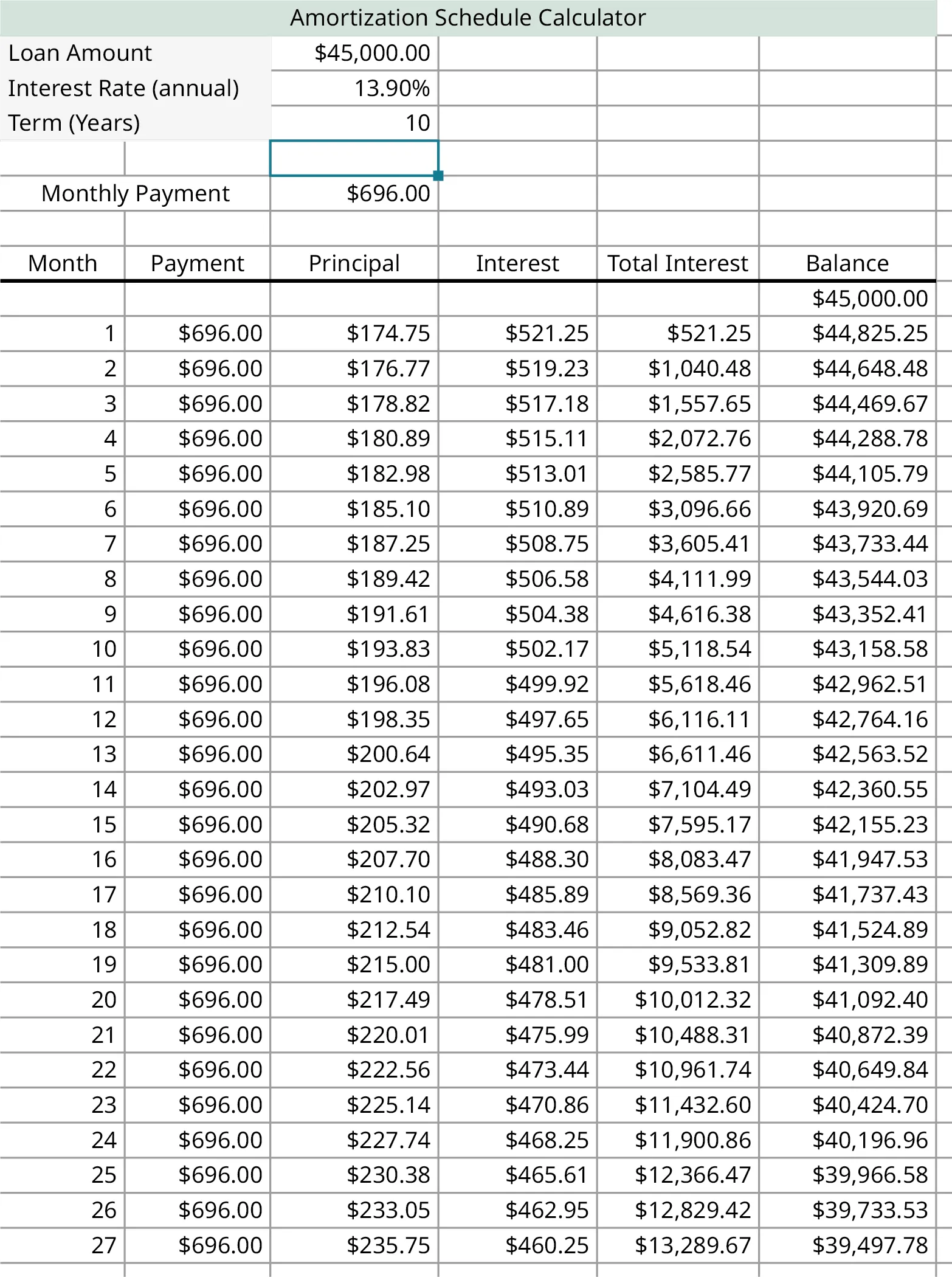### Section 6.8 Exercises

1 .
How do fixed interest rates and variable interest rates differ?
2 .
What is revolving credit?
3 .
What is loan amortization?
4 .
What are the two components of a loan payment?
5 .
Name three details that are presented on an amortization schedule.
For the following exercises, calculate the interest due for the monthly installment payment given the remaining principal and interest rate.
6 .
Remaining principal = $21,872.99, interest rate = 13.9% 7 . Remaining principal =$2,845.43, interest rate = 4.99%
8 .
Remaining principal = $78,913.76, interest rate = 2.9% 9 . Remaining principal =$6,445.22, interest rate = 5.65%
In the following exercises, calculate the monthly payment for the loan.
10 .
Principal = $8,600, annual interest rate = 6.75%, term is 5 years 11 . Principal =$19,400, annual interest rate = 2.25%, term is 6 years
12 .
Principal = $11,870, annual interest rate = 3.59%, term is 3 years 13 . Principal =$41,900, annual interest rate = 8.99%, term is 15 years
14 .
Principal = $26,150, annual interest rate = 11.1%, term is 7 years 15 . Principal =$46,350, annual interest rate = 2.9%, term is 6 years
16 .
Principal = $175,800, annual interest rate = 4.73%, term is 25 years 17 . Principal =$225,000, annual interest rate = 5.06%, term is 30 years
In the following exercises, use the amortization table below to answer the questions.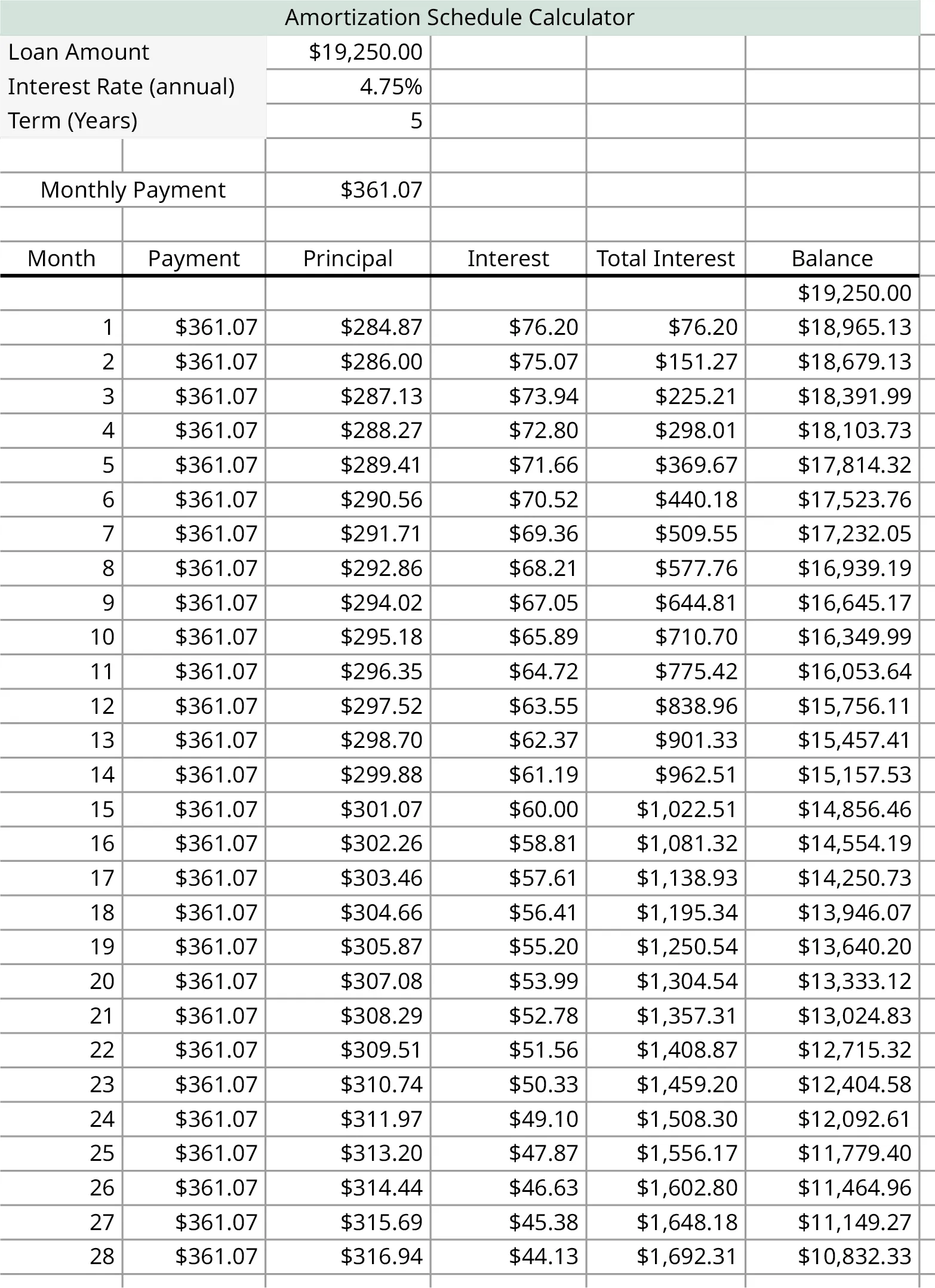18 .
What was the loan amount, or starting principal?
19 .
What was the interest rate?
20 .
What is the term of the loan?
21 .
What are the monthly payments?
22 .
What is the remaining principal after payment 17?
23 .
How much of payment 27 was for interest?
24 .
How much of payment 19 was for principal?
25 .
How much total interest was paid after payment 22?
In the following exercises, use the amortization table for payments 131–152 of a mortgage below to answer the questions.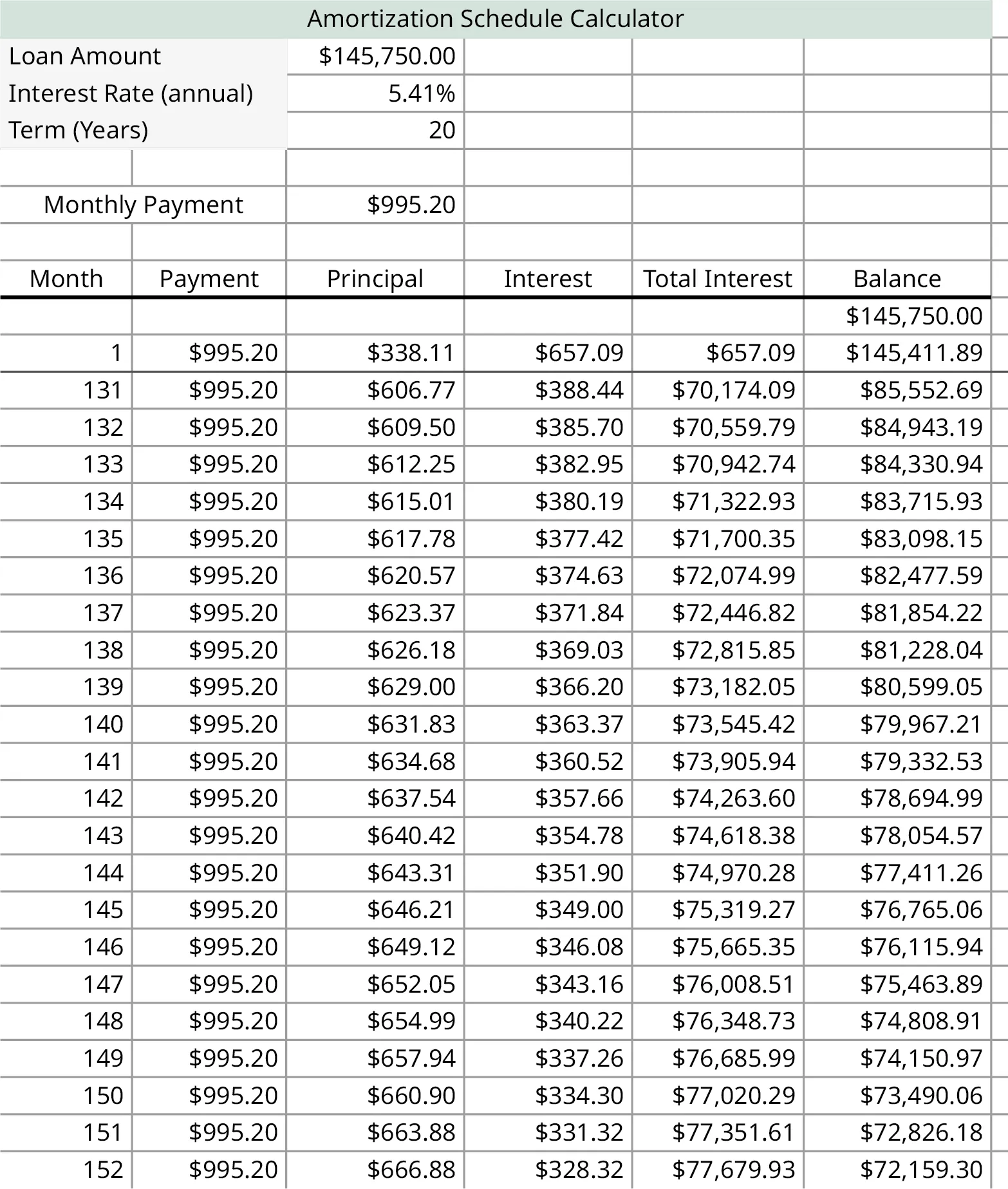26 .
What was the loan amount, or starting principal?
27 .
What was the interest rate?
28 .
What is the term of the loan?
29 .
What are the monthly payments?
30 .
What is the remaining principal after payment 140?
31 .
How much of the principal has been paid off after payment 151?
32 .
How much of payment 138 was for principal?
33 .
How much total interest was paid after payment 135?
In the following exercises find the cost to finance for the loan.
34 .
Total interest paid is $94,598.36, origination fee was$450, processing fee was $300, commission fee was$1,457.50.
35 .
Total interest paid was $3,209.34, origination fee was$100, processing fee was $200. 36 . Total interest paid was$8,295.50, fees were $875. 37 . Total interest paid was$56,114.90, origination fee was $1,230, and filing fee was$250.
38 .
Kylie takes out a $16,780 loan from her credit union for a new car. The loan’s term is 4 years with an interest rate of 6.77%. What are Kylie’s monthly payments? 39 . Crissy and Jonesy take out a$13,200 loan for repairs to their roof. The loan is for 10 years at 7.15% interest. What are their payments for this loan?
Below are two amortization tables. They are for loans with the same principal and interest rate, but different terms, the first a 20-year term and the second a 30-year term. The tables show the last payments of each loan. Use those two tables for the following exercises.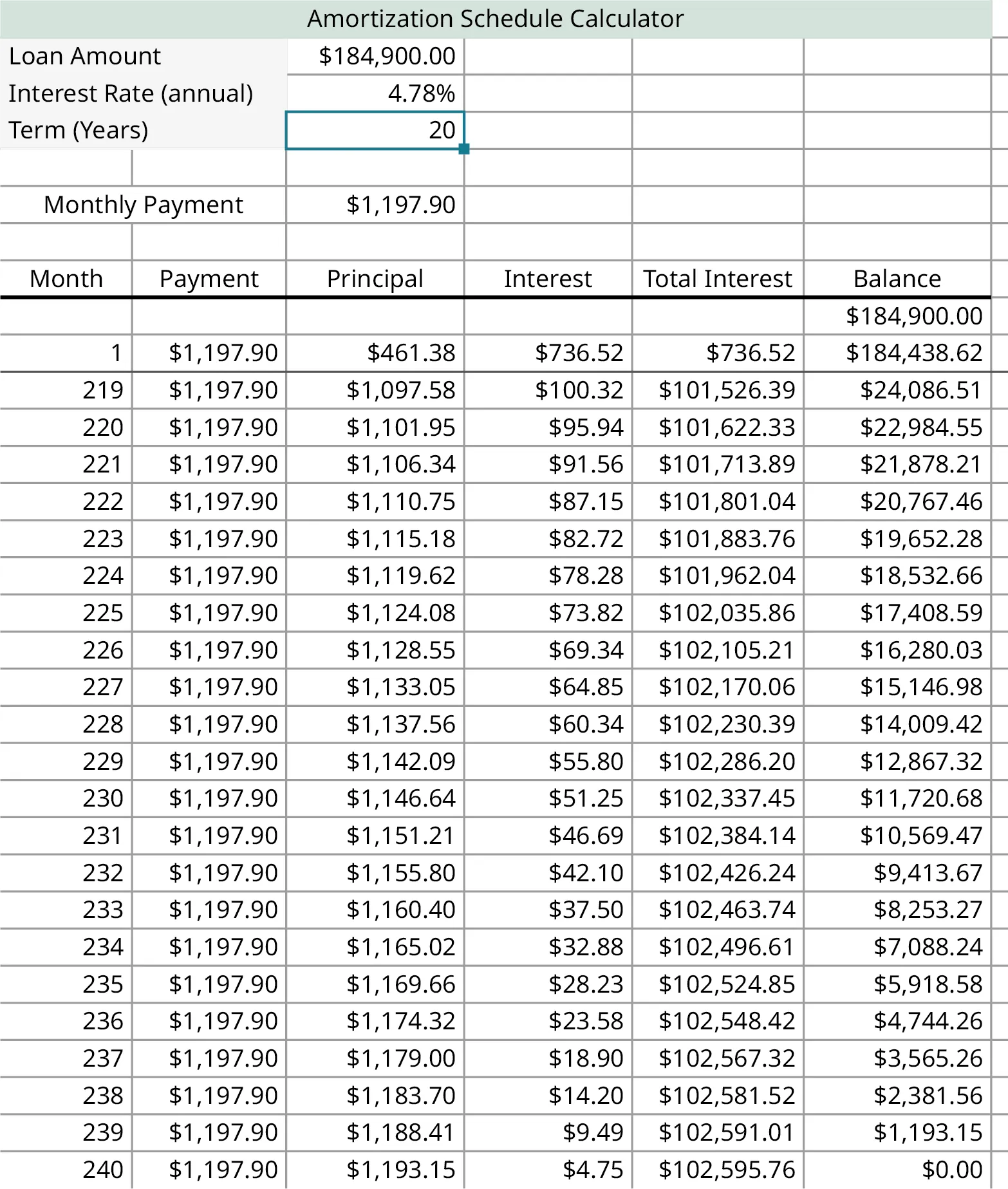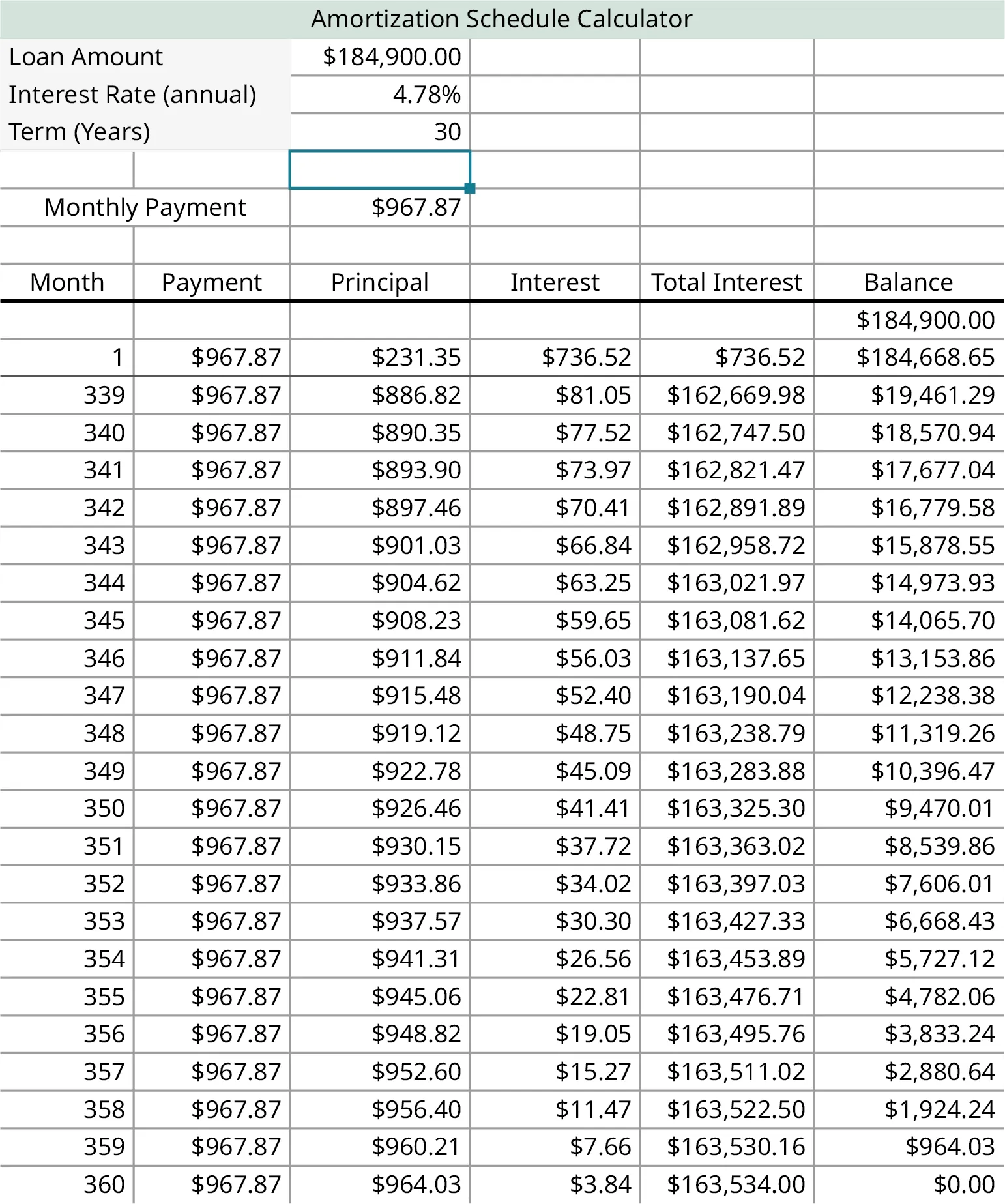40 .
How much total interest was paid for the 20-year loan? The 30-year loan? Calculate the difference.
41 .
What was the payment for the 20-year loan? The 30-year loan? Calculate the difference.
42 .
Compare the two loans. Why would the 30-year be preferable over the 20-year loan? Why would the 20-year loan be preferable to the 30-year loan?
In the following exercises, compare payments per month and interest rates.
43 .
A loan for $20,000 is taken out for 5 years. Find the payment is the interest rate is: 1. 2.9% 2. 3.9% 3. 4.9% 4. 5.9% 5. Did the payments increase by the same amount for each 1% jump in interest rate? Describe the pattern. 44 . A loan for$50,000 is taken out for 7 years. Find the payment is the interest rate is:
1. 3.5%
2. 4.5%
3. 5.5%
4. 6.5%
5. Did the payments increase by the same amount for each 1% jump in interest rate?
Order a print copy

As an Amazon Associate we earn from qualifying purchases.